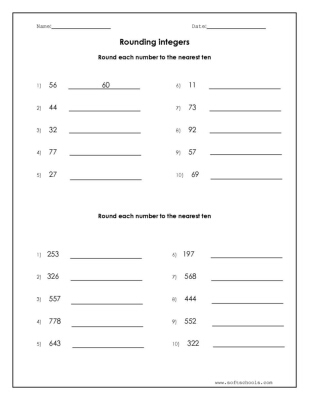# Round each number to the nearest ten

Round each number to the nearest ten
Round each number to the nearest tenRound each number to the nearest hundred
Round each number to the nearest ten
Round each number to the nearest tens and hundreds
Round to the Nearest Hundred
Round to the Nearest Ten
Round each number to the nearest hundred
Round each number to the nearest hundred
Round each number to the nearest tens and hundreds
Round each number to the nearest tens and hundreds
Round each number to the nearest ten